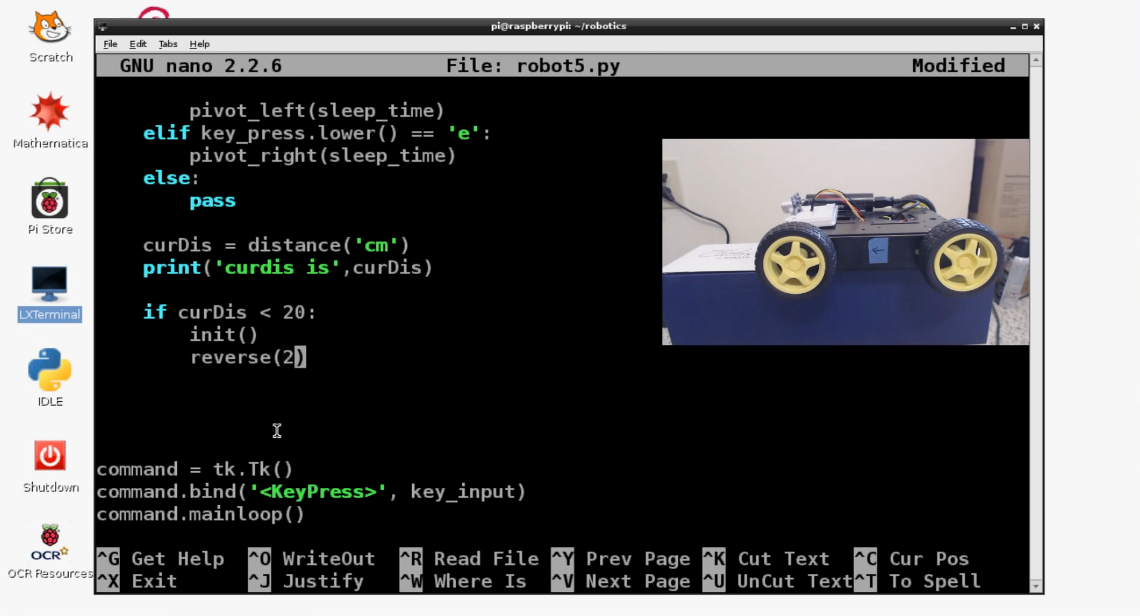## Autopilot and/or auto-correct

```import RPi.GPIO as gpio
import time
import sys
import Tkinter as tk
from sensor import distance

def init():
gpio.setmode(gpio.BOARD)
gpio.setup(7, gpio.OUT)
gpio.setup(11, gpio.OUT)
gpio.setup(13, gpio.OUT)
gpio.setup(15, gpio.OUT)

def forward(tf):
gpio.output(7, False)
gpio.output(11, True)
gpio.output(13, True)
gpio.output(15, False)
time.sleep(tf)
gpio.cleanup()

def reverse(tf):
gpio.output(7, True)
gpio.output(11, False)
gpio.output(13, False)
gpio.output(15, True)
time.sleep(tf)
gpio.cleanup()

def turn_left(tf):
gpio.output(7, True)
gpio.output(11, True)
gpio.output(13, True)
gpio.output(15, False)
time.sleep(tf)
gpio.cleanup()

def turn_right(tf):
gpio.output(7, False)
gpio.output(11, True)
gpio.output(13, False)
gpio.output(15, False)
time.sleep(tf)
gpio.cleanup()

def pivot_left(tf):
gpio.output(7, True)
gpio.output(11, False)
gpio.output(13, True)
gpio.output(15, False)
time.sleep(tf)
gpio.cleanup()

def pivot_right(tf):
gpio.output(7, False)
gpio.output(11, True)
gpio.output(13, False)
gpio.output(15, True)
time.sleep(tf)
gpio.cleanup()

def key_input(event):
init()
print 'Key:', event.char
key_press = event.char
sleep_time = 0.030

if key_press.lower() == 'w':
forward(sleep_time)
elif key_press.lower() == 's':
reverse(sleep_time)
elif key_press.lower() == 'a':
turn_left(sleep_time)
elif key_press.lower() == 'd':
turn_right(sleep_time)
elif key_press.lower() == 'q':
pivot_left(sleep_time)
elif key_press.lower() == 'e':
pivot_right(sleep_time)
else:
pass

curDis = distance('cm')
print('curdis is',curDis)

if curDis < 20:
init()
reverse(2)

command = tk.Tk()
command.bind('<KeyPress>', key_input)
command.mainloop()

```

Now that we understand the distance sensor, and we've got this neat little sensor module written up, let's incorporate it into our car

First, let's use it as a sort of auto-pilot. Some cars today already will apply your brakes for you if they sense you are getting too close to another car, this is sort of like that.

All we really need to do is do some distance checks within our tkinter key-logging loop. If the distance check yields a distance less than an acceptable distance, then stop. Easy enough!

Not only will we stop here, but we're also going to apply an instantaneous reversal.

The next tutorial:• Robotics with the Raspberry Pi

• Programming GPIO example

• Running GPIO

• Building Autonomous / RC car intro

• Supplies needed

• Motor Control

• Connecting the four motors

• Forward and Reverse

• Turning

• Pivoting

• User Control

• Remotely controlling the car

• Adding a distance sensor (HC-SR04)

• Programming with the distance sensor

• Autopilot and/or auto-correct
• Autonomous Beginnings

• Testing Autonomous Code

• Streaming video example one

• Less latency streaming option